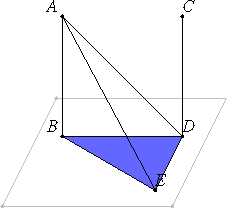# Proposition 6

If two straight lines are at right angles to the same plane, then the straight lines are parallel.

Let the two straight lines AB and CD be at right angles to the plane of reference.

I say that AB is parallel to CD.

Let them meet the plane of reference at the points B and D.Join the straight line BD. Draw DE in the plane of reference at right angles to BD, and make DE equal to AB.

XI.Def.3

Now, since AB is at right angles to the plane of reference, it also makes right angles with all the straight lines which meet it and lie in the plane of reference.

But each of the straight lines BD and BE lies in the plane of reference and meets AB, therefore each of the angles ABD and ABE is right. For the same reason each of the angles CDB and CDE is also right.

I.4

And since AB equals DE, and BD is common, therefore the two sides AB and BD equal the two sides ED and DB. And they include right angles, therefore the base AD equals the base BE

I.8

And, since AB equals DE while AD equals BE, the two sides AB and BE equal the two sides ED and DA, and AE is their common base, therefore the angle ABE equals the angle EDA.

But the angle ABE is right, therefore the angle EDA is also right. Therefore ED is at right angles to DA.

XI.5

But it is also at right angles to each of the straight lines BD and DC, therefore ED is set up at right angles to the three straight lines BD, DA, and DC at their intersection. Therefore the three straight lines BD, DA, and DC lie in one plane.

XI.2

But in whatever plane DB and DA lie, AB also lies, for every triangle lies in one plane.

Therefore the straight lines AB, BD, and DC lie in one plane. And each of the angles ABD and BDC is right, therefore AB is parallel to CD.

I.28

Therefore, if two straight lines are at right angles to the same plane, then the straight lines are parallel.

Q.E.D.

## Guide

Euclid does not consider the possibility that the two lines meet the plane at one point, but that possibility can easily be eliminated. Indeed, that is the statement of proposition XI.13 which, therefore, should precede this one.

A converse of this proposition is XI.8.

#### Use of this proposition

This proposition is used in the proofs of propositions XII.17, XIII.16, and XIII.17.